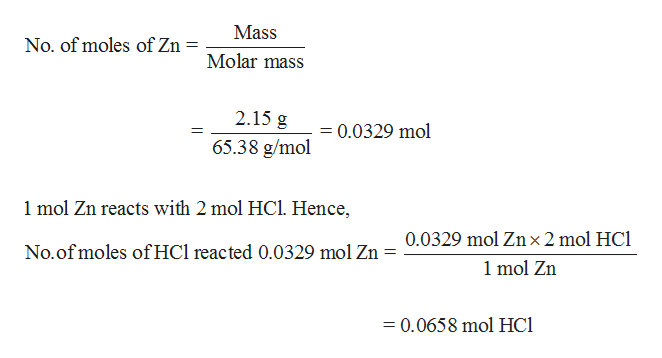# Zinc reacts with hydrochloric acid according to the reaction equation Zn(s)+2HCl(aq)⟶ZnCl2(aq)+H2(g) How many milliliters of 5.50 M HCl(aq) are required to react with 2.15 g Zn(s)?

Question
132 views

Zinc reacts with hydrochloric acid according to the reaction equation

Zn(s)+2HCl(aq)⟶ZnCl2(aq)+H2(g)

How many milliliters of 5.50 M HCl(aq) are required to react with 2.15 g Zn(s)?

check_circle

Step 1

Number of moles of HCl needed to react with...help_outlineImage TranscriptioncloseMass No. of moles of Zn Molar mass 2.15 g =0.0329 mol 65.38 g/mol 1 mol Zn reacts with 2 mol HCl. Hence, 0.0329 mol Zn x 2 mol HCl No.of moles of HCl reacted 0.0329 mol Zn = 1 mol Zn =0.0658 mol HCl fullscreen

### Want to see the full answer?

See Solution

#### Want to see this answer and more?

Solutions are written by subject experts who are available 24/7. Questions are typically answered within 1 hour.*

See Solution
*Response times may vary by subject and question.
Tagged in

### Quantitative analysis